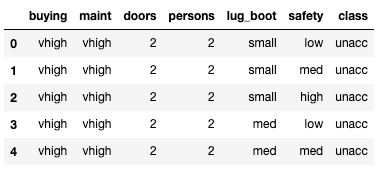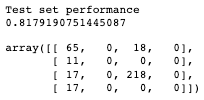top of page
Search
•Sai Geetha M N

# Decision Trees through an Example

We have so far seen what decision trees are, why we need them, what are certain measures that help in creating a decision tree and how features are selected for a split at a particular node.

There are a few more concepts that would help in using decision trees practically. However, I felt sharing a good piece of code to show how they are built, would help in taking a break from too much theory.

To appreciate or understand this, please go through these posts before you start on this example.

Decision Trees - An Introduction

#### Example Data

I have downloaded the data from Kaggle - called the "Car Evaluation Data Set" for this example. This uses the overall price of the car, the maintenance cost, the number of doors, the number of people it can accommodate, the boot capacity and safety to decide whether that car is acceptable, good or very good or totally unacceptable.

NOTE: The data and code for this article is fully available in my Github repo as a jupyter notebook. You can look at it in detail and execute it to understand the complete flow.

#### Initial Steps: Data Understanding and Preparation

In the notebook, the first step is to read and understand the data. The code there is simple and straightforward and hence not walking through that here.

The next step is to analyse and prepare data.

The data has no missing values. Also, it has all categorical data and hence no question of having outliers. Therefore, we move straight into splitting the data for train and test, following which, categorical encoding of data is done.

Create the feature set X and the target variable y

```X = df.drop(['class'], axis=1)
y = df['class']```

Split the data set into train and test data sets:

```from sklearn.model_selection import train_test_split

X_train, X_test, y_train, y_test = train_test_split(X, y, test_size = 0.20, random_state = 42)```

Now, the categorical encoder is used to convert all the features into ordinal variables

```encoder = ce.OrdinalEncoder(cols=['buying', 'maint', 'doors', 'persons', 'lug_boot', 'safety'])

X_train = encoder.fit_transform(X_train)

X_test = encoder.transform(X_test)```

After encoding, the features' data has changed like this:compared to the original data which was like this:Please read this post on Types of Variables, if you want to understand more about types of variables like categorical and numerical etc.

If the variables are ordinal in nature i.e. they have an inherent order in them, like the cost is low, medium, high, or very high, has an inherent order in it. Then, we use "OrdinalEncoder" for encoding the same.

### Decision Tree Modeling

Now that the data is ready, we can start modelling the data.

```from sklearn.tree import DecisionTreeClassifier

dt = DecisionTreeClassifier(max_depth=3)
dt.fit(X_train, y_train)```

With these three lines of code, the model is ready!!

However, there are a lot of parameters that need to be tuned or controlled to get a useful decision tree model. Here, I have controlled only the depth of the model creation to 3. Some of the other parameters that can be tuned are max_features, max_leaf_nodes, min_impurity_decrease and so on. The default impurity criterion is 'gini' and hence gini index as described in Decision Trees - Homogeneity Measures is used for calculating the impurity of a node.

Following the creation of the model, you would want to visualize the model.

This piece of code provides a very basic visualisation.

`tree.plot_tree(dt.fit(X_train, y_train))`

However, I have tried to visualise the model using graphviz and pydotplus libraries. Installation of these could be done in your python environment using 'pip install' or you conda environment using 'conda install'.

```pip install pydotplus
pip install python-graphviz
# or
conda install pydotplus
conda install python-graphviz
# or
# if you want to manage some SSL errors, do this:
pip3 install --trusted-host pypi.org --trusted-host files.pythonhosted.org graphviz
pip install --trusted-host pypi.org --trusted-host files.pythonhosted.org pydotplus```

The graph that is obtained gives a lot of visual information about the measure that was used to check the homogeneity of the nodes and the features based on which a split has been done.

I am not getting into the code that draws the graph as it is standard boilerplate code that will work for any graphviz object given out by sklearn modules#### Interpretation of the model

The tree shows that the first check is based on safety. Then, it checks on the number of persons followed by maintenance cost. Since we see the max_level is 3 it stops there. And it keeps separating out groups with a Gini of 0.0 meaning they are completely pure groups.

The root node Gini is 0.452. Meaning it is not a completely pure node. The first check done is whether safety <= 2.5. This node started with a sample size of 1382.

value = [301, 58, 975, 48] tells us how many of each category of cars are there in this node, the categories being acceptable, good, unacceptable and very good.

And the 'class' of course tells us whether this node is classified into which category. The class that occurs the most in the node dictates the class of the node.

At every level, you can see that based on some criteria, the pure node consists of only unacceptable cars and hence a Gini index of 0.0

#### Validating the model

Having visualized the model, now we want to validate if this model works well on unseen data (here it is the test data).

So, predict the class of the test data using the fitted model

`y_test_pred = dt.predict(X_test)`

Then you check the accuracy and the confusion matrix

```accuracy_score(y_test, y_test_pred)
confusion_matrix(y_test, y_test_pred)```

You do the same for train data also, to compare the two scores and see if the model has overfitted.

The output obtained for test data is:This says the accuracy of prediction on the test data is 81.79% which is a decent accuracy score. The confusion matrix is a 4 x 4 matrix in this case as it is a case of multi-class classification problem. Since we have 4 classes of classification, it is a 4 x 4 matrix.

How to interpret the confusion matrix is something that I will reserve for another post. In short, the confusion matrix gives a count of true positives, true negatives, false positives known as type 1 errors and false negatives known as type 2 errors.

#### Conclusion

The train data set accuracy score is 80.24% and that of the test data set is 81.79%. They are very close to each other and hence we can conclude that a good model has been created by the sklearn's DecisionTreeClassifier with a max_depth parameter of 3.

The model creation is a set of simple steps. However, hyperparameter tuning and getting a good model is an art that is learnt with experimentation and experience over time. Also, the data in real life is never so well prepared upfront. it has to be cleaned and prepared to be fed into a library that creates the model.